Essential Math for Data Science
Do you want more math for data science and machine learning? I just released my book "Essential Math for Data Science"🎉.

I’m glad to announce a few updates concerning my book Essential Math for Data Science.

• First, I changed the structure of the book: a first chapter on basic algebra has been removed. Part of old chapter 02 has been merged in the linear algebra part.

• I restructured the table of content: I removed some content about very basic math (like what is an equation or a function) to have more space to cover slighly more advanced contents. The part Statistics and Probability is now at the beginning of the book (just after a first part on Calculus). Have a look at the new TOC below to have more details.

• There is now build hands-on projects for each chapter. Hands-on projects are sections where you can apply the math you just learned to a practical machine learning problem (like gradient descent or regularization, for instance). The difficulty of math and code in each of these hands-on project is variable, so you should find something at the right point of your learning curve.

Here is the table of content. Click on the chapters to see what’s inside.

### Table of Content

• expand_more 01. How to use this Book

#### PART 1. Calculus

Machine learning and data science require some experience with calculus. You’ll be introduced here to derivatives and integrals and how they are useful in machine learning and data science.

• expand_more 02. Calculus: Derivatives and Integrals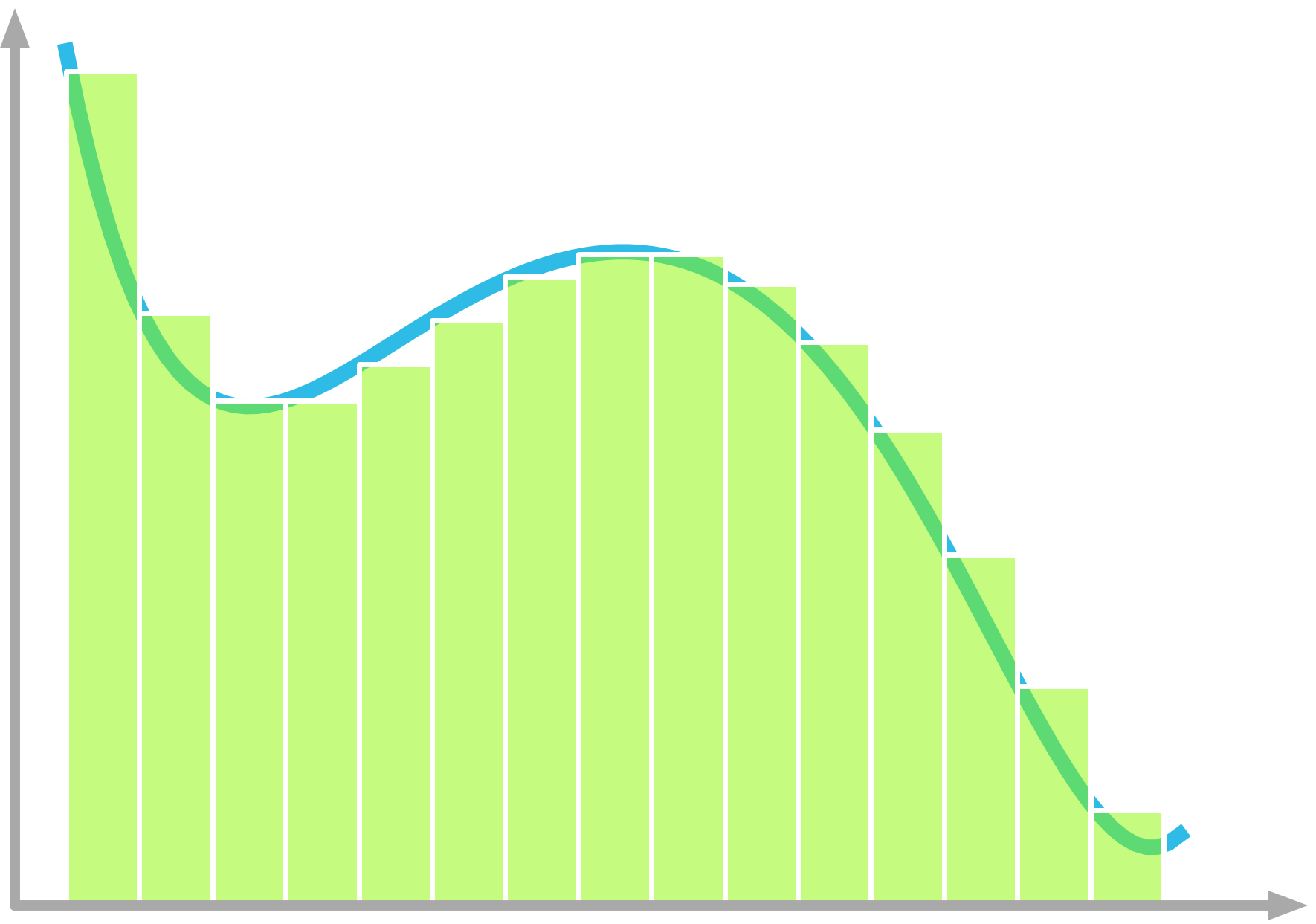Area under the curve
• expand_more 2.1 Derivatives

2.1.1 Introduction

2.1.2 Mathematical Definition of Derivatives

2.1.3 Derivatives of Linear And Nonlinear Functions

2.1.4 Derivative Rules

• expand_more 2.2 Integrals And Area Under The Curve

2.2.1 Example

2.2.2 Riemann Sum

2.2.3 Mathematical Definition

• build2.3 Hands-On Project: Gradient Descent

2.3.1 Cost function

2.3.2 Derivative of the Cost Function

2.3.4 MSE Cost Function With Two Parameters

#### PART 2. Statistics and Probability

In machine learning and data science, probability and statistics are used to deal with uncertainty. This uncertainty comes from various sources, from data collection to the process you’re trying to model itself. This part will introduce you to descriptive statistics, probability distributions, bayesian statistics and information theory.

• expand_more 03. Statistics and Probability Theory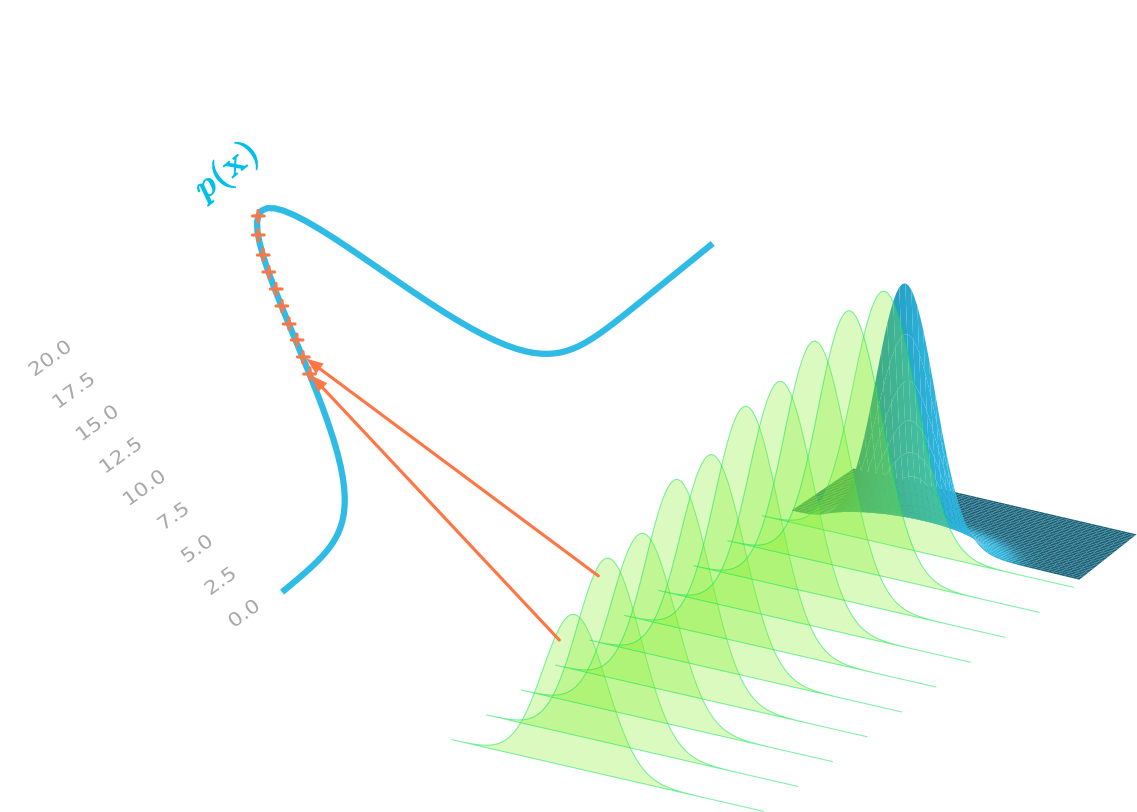Joint and Marginal Probability
• expand_more 3.1 Descriptive Statistics

3.1.1 Variance and Standard Deviation

3.1.2 Covariance and Correlation

3.1.3 Covariance Matrix

• expand_more 3.2 Random Variables

3.2.1 Definitions and Notation

3.2.2 Discrete and Continuous Random Variables

• expand_more 3.3 Probability Distributions

3.3.1 Probability Mass Functions

3.3.2 Probability Density Functions

2.3.4 MSE Cost Function With Two Parameters

• expand_more 3.4 Joint, Marginal, and Conditional Probability

3.4.1 Joint Probability

3.4.2 Marginal Probability

3.4.3 Conditional Probability

• expand_more 3.5 Cumulative Distribution Functions
• expand_more 3.6 Expectation and Variance of Random Variables

3.6.1 Discrete Random Variables

3.6.2 Continuous Random Variables

3.6.3 Variance of Random Variables

• build3.7 Hands-On Project: The Central Limit Theorem

3.7.1 Continuous Distribution

3.7.2 Discrete Distribution

• expand_more 04. Common Probability Distributions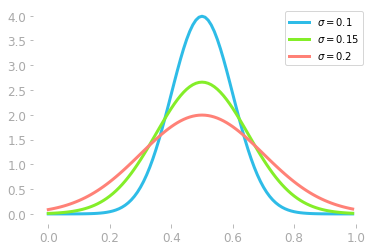Gaussian Distributions
• expand_more 4.1 Uniform Distribution
• expand_more 4.2 Gaussian distribution

4.2.1 Formula

4.2.2 Parameters

4.2.3 Requirements

• expand_more 4.3 Bernoulli Distribution
• expand_more 4.4 Binomial Distribution

4.4.1 Description

4.4.2 Graphical Representation

• expand_more 4.5 Poisson Distribution

4.5.1 Mathematical Definition

4.5.2 Example

• expand_more 4.6 Exponential Distribution

4.6.1 Derivation from the Poisson Distribution

4.6.2 Effect of λ

• build4.7 Hands-on Project: Waiting for the Bus
• expand_more 05. Bayesian Statistics and Information Theory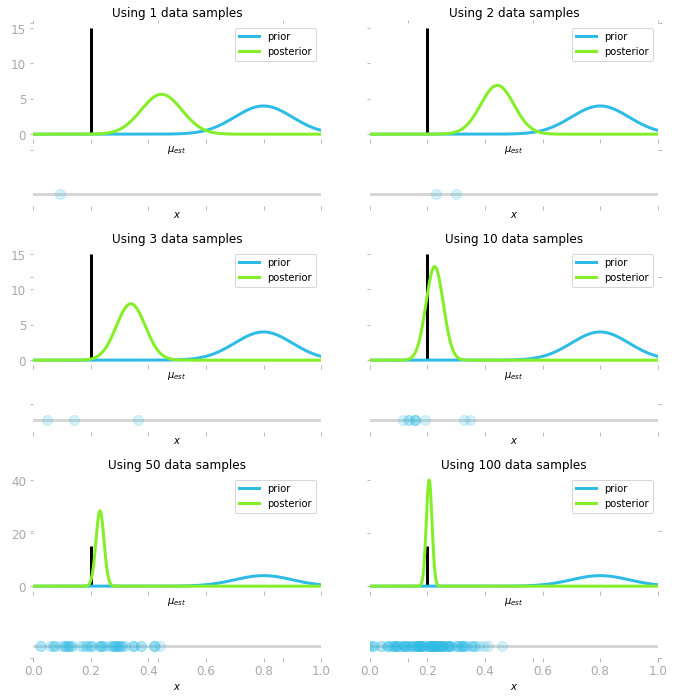Bayesian Inference
• expand_more 5.1 Bayes’ Theorem

5.1.1 Mathematical Formulation

5.1.2 Example

5.1.3 Bayesian Interpretation

5.1.4 Bayes’ Theorem with Distributions

• expand_more 5.2 Likelihood

5.2.1 Introduction and Notation

5.2.2 Finding the Parameters of the Distribution

5.2.3 Maximum Likelihood Estimation

• expand_more 5.3 Information Theory

5.3.1 Shannon Information

5.3.2 Entropy

5.3.3 Cross Entropy

5.3.4 Kullback-Leibler Divergence (KL Divergence)

• build5.4 Hands-On Project: Bayesian Inference

5.4.2 Project

#### PART 3. Linear Algebra

Linear algebra is the core of many machine learning algorithms. The great news is that you don’t need to be able to code these algorithms yourself. It is more likely that you’ll use a great Python library instead. However, to be able to choose the right model for the right job, or to debug a broken machine learning pipeline, it is crucial to have enough understanding of what’s under the hood. The goal of this part is to give you enough understanding and intuition about the major concepts of linear algebra used in machine and data science. It is designed to be accessible, even if you never studied linear algebra.

• expand_more 06. Scalars and Vectors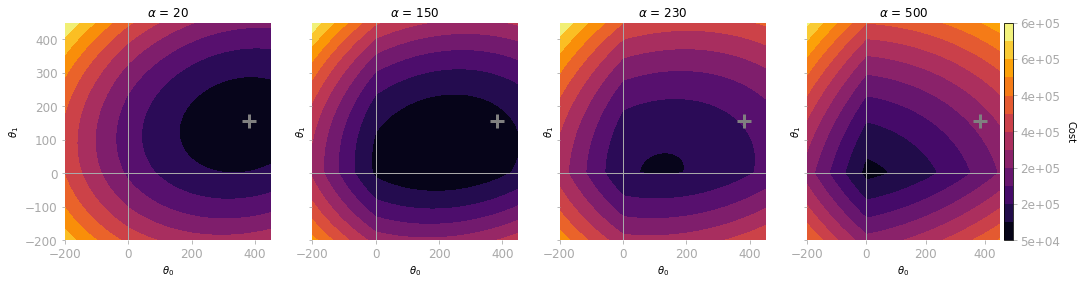L1 Regularization. Effect of Lambda.
• expand_more 6.1 What Vectors are?

6.1.1 Geometric and Coordinate Vectors

6.1.2 Vector Spaces

6.1.3 Special Vectors

• expand_more 6.2 Operations and Manipulations on Vectors

6.2.1 Scalar Multiplication

6.2.3 Transposition

• expand_more 6.3 Norms

6.3.1 Definitions

6.3.2 Common Vector Norms

6.3.3 Norm Representations

• expand_more 6.4 The Dot Product

6.4.1 Definition

6.4.2 Geometric interpretation: Projections

6.4.3 Properties

• build6.5 Hands-on Project: Regularization

6.5.1 Introduction

6.5.2 Effect of Regularization on Polynomial Regression

6.5.3 Differences between $L^1$ and $L^2$ Regularization

• expand_more 07. Matrices and Tensors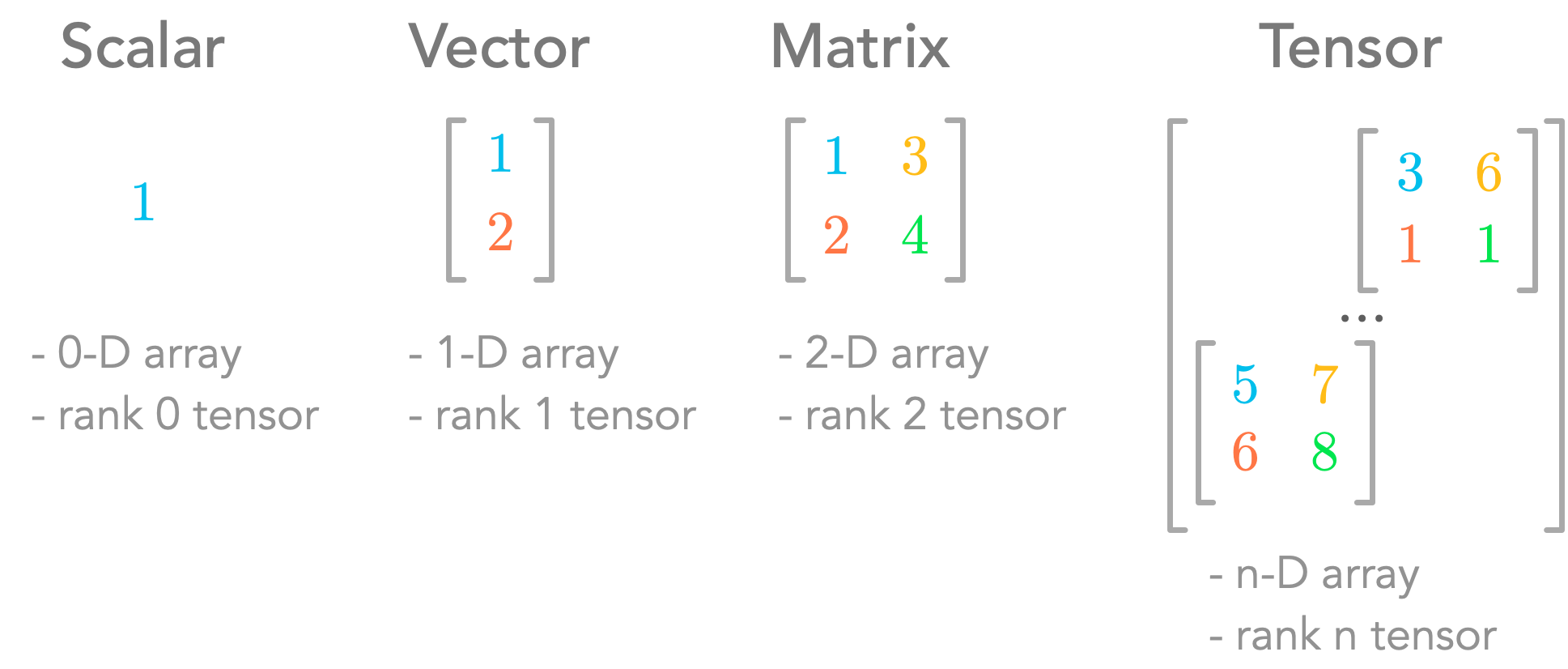Scalars, vectors, matrices and tensors
• expand_more 7.1 Introduction

7.1.1 Matrix Notation

7.1.2 Shapes

7.1.3 Indexing

7.1.4 Main Diagonal

7.1.5 Tensors

7.1.6 Frobenius Norm

• expand_more 7.2 Operations and Manipulations on Matrices

7.2.2 Transposition

• expand_more 7.3 Matrix Product

7.3.1 Matrices with Vectors

7.3.2 Matrices Product

7.3.3 Transpose of a Matrix Product

• expand_more 7.4 Special Matrices

7.4.1 Square Matrices

7.4.2 Diagonal Matrices

7.4.3 Identity Matrices

7.4.4 Inverse Matrices

7.4.5 Orthogonal Matrices

7.4.6 Symmetric Matrices

7.4.7 Triangular Matrices

• build7.5 Hands-on Project: Image Classifier

7.5.1 Images as Multi-dimensional Arrays

7.5.2 Data Preparation

• expand_more 08. Span, Linear Dependency, and Space Transformation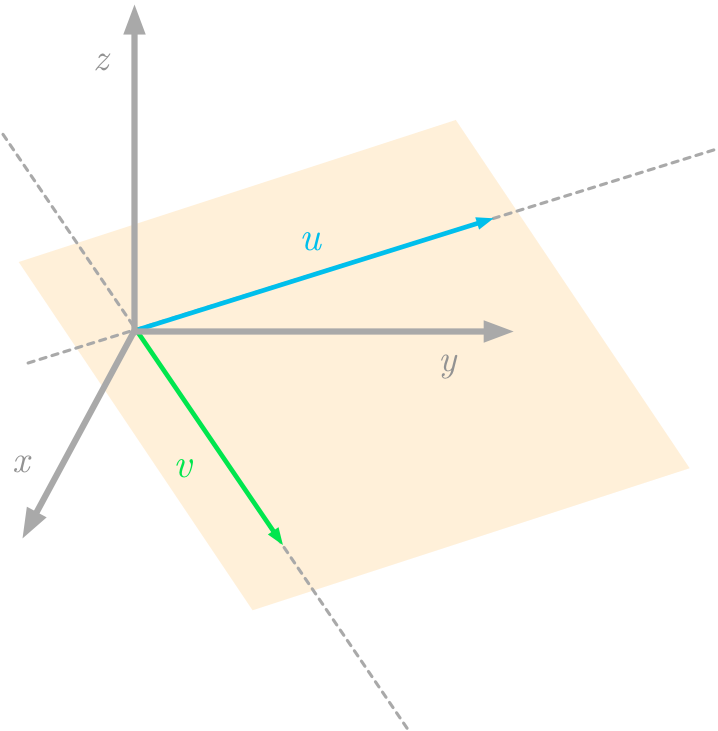All linear Combinations of two vectors
• expand_more 8.1 Linear Transformations

8.1.1 Intuition

8.1.2 Linear Transformations as Vectors and Matrices

8.1.3 Geometric Interpretation

8.1.4 Special Cases

• expand_more 8.2 Linear combination

8.2.1 Intuition

8.2.2 All combinations of vectors

8.2.3 Span

• expand_more 8.3 Subspaces

8.3.1 Definitions

8.3.2 Subspaces of a Matrix

• expand_more 8.4 Linear dependency

8.4.1 Geometric Interpretation

8.4.2 Matrix View

• expand_more 8.5 Basis

8.5.1 Definitions

8.5.2 Linear Combination of Basis Vectors

8.5.3 Other Bases

• expand_more 8.6 Special Characteristics

8.6.1 Rank

8.6.2 Trace

8.6.3 Determinant

• build8.7 Hands-On Project: Span
• expand_more 09. Systems of Linear Equations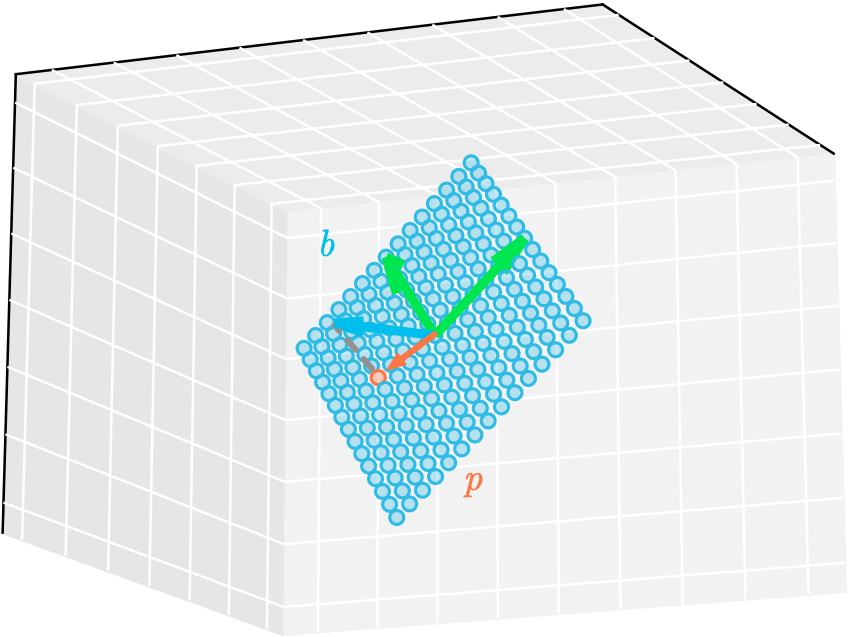Projection of a vector onto a plane
• expand_more 9.1 System of linear equations

9.1.1 Row Picture

9.1.2 Column Picture

9.1.3 Number of Solutions

9.1.4 Representation of Linear Equations With Matrices

• expand_more 9.2 System Shape

9.2.1 Overdetermined Systems of Equations

9.2.2 Underdetermined Systems of Equations

• expand_more 9.3 Projections

9.3.1 Solving Systems of Equations

9.3.2 Projections to Approximate Unsolvable Systems

9.3.3 Projections Onto a Line

9.3.4 Projections Onto a Plane

• build9.4 Hands-on Project: Linear Regression Using Least Approximation

9.4.1 Linear Regression Using the Normal Equation

9.4.2 Relationship Between Least Squares and the Normal Equation

• expand_more 10. Eigenvectors and Eigenvalues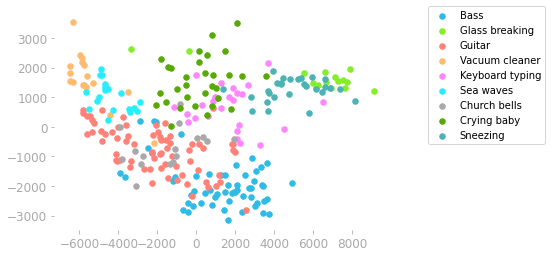Principal Component Analysis on audio samples.
• expand_more 10.1 Eigenvectors and Linear Transformations
• expand_more 10.2 Change of Basis

10.2.1 Linear Combinations of the Basis Vectors

10.2.2 The Change of Basis Matrix

10.2.3 Example: Changing the Basis of a Vector

• expand_more 10.3 Linear Transformations in Different Bases

10.3.1 Transformation Matrix

10.3.2 Transformation Matrix in Another Basis

10.3.3 Interpretation

• expand_more 10.4 Eigendecomposition

10.4.1 First Step: Change of Basis

10.4.2 Eigenvectors and Eigenvalues

10.4.3 Diagonalization

10.4.4 Eigendecomposition of Symmetric Matrices

• build10.5 Hands-On Project: Principal Component Analysis

10.5.1 Under the Hood

10.5.2 Making Sense of Audio

• expand_more 11. Singular Value Decomposition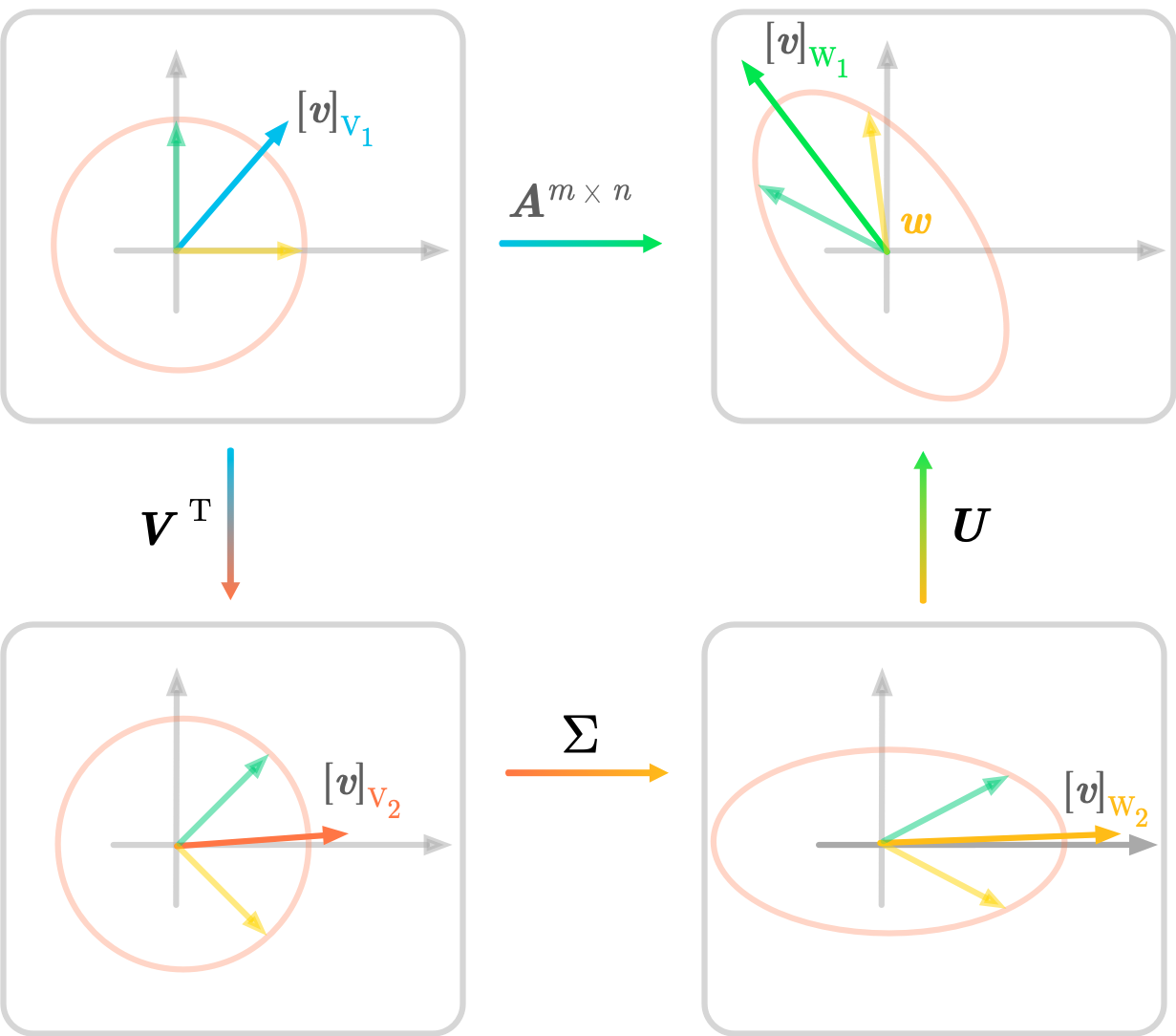SVD Geometry
• expand_more 11.1 Nonsquare Matrices

11.1.1 Different Input and Output Spaces

11.1.2 Specifying the Bases

11.1.3 Eigendecomposition is Only for Square Matrices

• expand_more 11.2 Expression of the SVD

11.2.1 From Eigendecomposition to SVD

11.2.2 Singular Vectors and Singular Values

11.2.3 Finding the Singular Vectors and the Singular Values

11.2.4 Summary

• expand_more 11.3 Geometry of the SVD

11.3.1 Two-Dimensional Example

11.3.2 Comparison with Eigendecomposition

11.3.3 Three-Dimensional Example

11.3.4 Summary

• expand_more 11.4 Low-Rank Matrix Approximation

11.4.1 Full SVD, Thin SVD and Truncated SVD

11.4.2 Decomposition into Rank One Matrices

• build 11.5 Hands-On Project: Image Compression

I hope that you’ll find this content useful! Feel free to contact me if you have any question, request, or feedback!

Essential Math for Data Science
Do you want more math for data science and machine learning? I just released my book "Essential Math for Data Science"🎉.